Get inspired by the success stories of our students in IIT JAM MS, ISI  MStat, CMI MSc DS.  Learn More

# Inverse Uniform Distribution | ISI MStat 2007 PSB Problem 4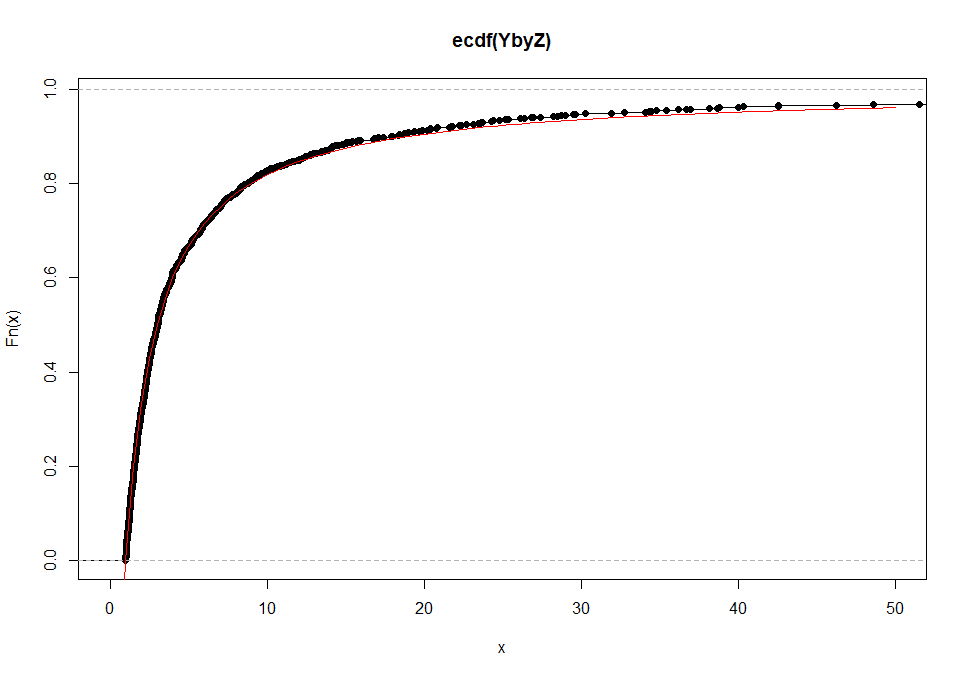This problem is an interesting application of the inverse uniform distribution family, which has infinite mean. This problem is from ISI MStat 2007. The problem is verified by simulation.

## Problem

The unit interval (0,1) is divided into two sub-intervals by picking a point at random from inside the interval. Denoting by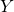andthe
lengths of the long and the shorter sub-intervals respectively show that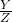does not have a finite expectation.

This is the 4th Problem ISI MStat 2008. Enjoy it.

### Prerequisites

• Distribution Function
• Distribution Function of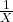in terms of the Distribution Function of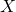.

## Solution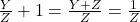, whereis the shorter length of the broken stick.

So,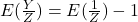.

Let's try to find the distribution of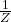.

Let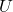~ Unif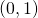whcih denotes the random uniform cut.

The shorter stick of length smaller than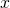can be achieved if the stick is cut either beforeor it is cut after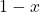.

Observe that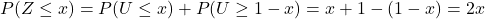. This answer is natural since, the total valid length is.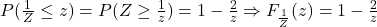if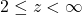.

Therefore,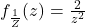if.

Hence,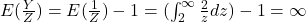## Simulation and Verification

Exercise: Prove that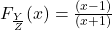if.

 u = runif(1000,0,1)
w = 1 - u
Z = pmin(u,w)
Y = pmax(u,w)
YbyZ = Y/Z
plot(ecdf(YbyZ), xlim = c(0,50))
x = seq(0, 50, 0.01)
curve((x - 1)/(x+1), from = 0, col = "red", add = TRUE)

The Mean moves really slowly to infinity ~ logx. Hence it is really hard to show it is going to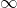. Also, the probability of occurrence of high value is almost 0. Hence, it really hard to show my simulation that the mean is. But, we can show that the mean of the maximum values is really large.

v = rep(0,200)
m = NULL
for ( i in 1:200)
{
#v[i] = 100*i
u = runif(10000,0,1)
w = 1 - u
Z = pmin(u,w)
Y = pmax(u,w)
YbyZ = Y/Z
m = c(m, max(YbyZ))
}
mean(m) = 79079.43

Beware of the simulation, it can be totally counterintuitive. This is really enjoyable though.

Stay Tuned!

This problem is an interesting application of the inverse uniform distribution family, which has infinite mean. This problem is from ISI MStat 2007. The problem is verified by simulation.

## Problem

The unit interval (0,1) is divided into two sub-intervals by picking a point at random from inside the interval. Denoting byandthe
lengths of the long and the shorter sub-intervals respectively show thatdoes not have a finite expectation.

This is the 4th Problem ISI MStat 2008. Enjoy it.

### Prerequisites

• Distribution Function
• Distribution Function ofin terms of the Distribution Function of.

## Solution, whereis the shorter length of the broken stick.

So,.

Let's try to find the distribution of.

Let~ Unifwhcih denotes the random uniform cut.

The shorter stick of length smaller thancan be achieved if the stick is cut either beforeor it is cut after.

Observe that. This answer is natural since, the total valid length is.if.

Therefore,if.

Hence,## Simulation and Verification

Exercise: Prove thatif.

 u = runif(1000,0,1)
w = 1 - u
Z = pmin(u,w)
Y = pmax(u,w)
YbyZ = Y/Z
plot(ecdf(YbyZ), xlim = c(0,50))
x = seq(0, 50, 0.01)
curve((x - 1)/(x+1), from = 0, col = "red", add = TRUE)

The Mean moves really slowly to infinity ~ logx. Hence it is really hard to show it is going to. Also, the probability of occurrence of high value is almost 0. Hence, it really hard to show my simulation that the mean is. But, we can show that the mean of the maximum values is really large.

v = rep(0,200)
m = NULL
for ( i in 1:200)
{
#v[i] = 100*i
u = runif(10000,0,1)
w = 1 - u
Z = pmin(u,w)
Y = pmax(u,w)
YbyZ = Y/Z
m = c(m, max(YbyZ))
}
mean(m) = 79079.43

Beware of the simulation, it can be totally counterintuitive. This is really enjoyable though.

Stay Tuned!

This site uses Akismet to reduce spam. Learn how your comment data is processed.

### Knowledge Partner